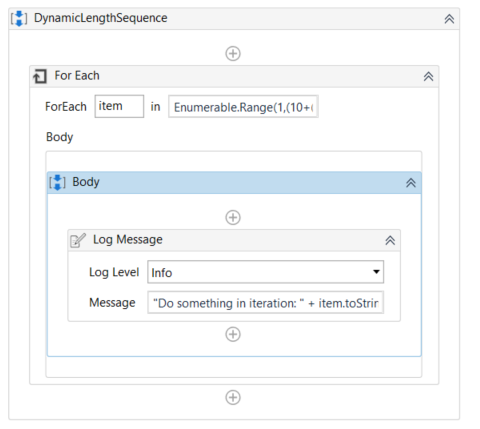# Hot key

Hello Ram,
I am developing a bot where i am in situation where i need to perform a specific activity for a (10+2 x) number of times, where x is a variable.
For example i need to send hotkey tab , when x=1 it should be like (10+2
1)12times
x=2 it should be like (10+2*2)14times

Thanks,
Vardhan

@Vishnu_gadikota all the variables are int32
pass value of x
Assign a counter variable…=1 ----a
add a while …give condition as a<=b
use send hotkey inside while ----
increase counter by 1 i.e a=a+1 after using send hotkey

3 Likes

with the help of Enumerable.Range we can do with less modelling steps compaired to an approach with do whilethe statement: Enumerable.Range(1,(10+(2*Factor)))

Find demo here:
DynamicLengthSequence.xaml (5.1 KB)

4 Likes

hello,
how to pass value of x

just use an assign activity
leftside: Factor
rightside: the x as Int32

1 Like